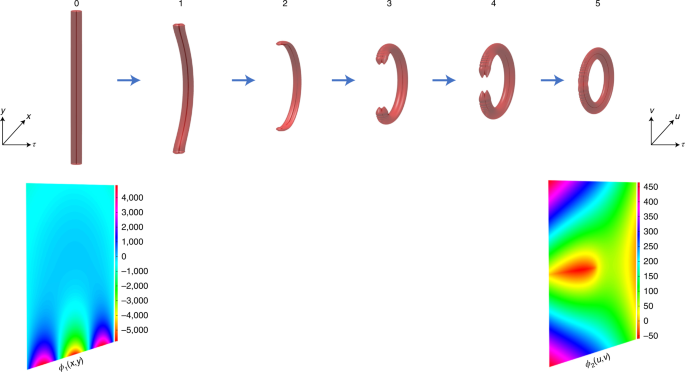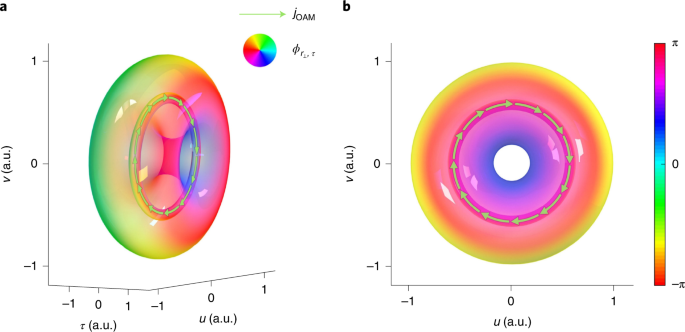## Main

Toroidal vortices, also known as vortex rings, are intriguing propagating ring-shaped structures with whirling disturbances rotating about the ring. Toroidal vortices are not uncommon in nature and science. Indeed, aquarium visitors are often amazed at how dolphins master creating and playing with bubble rings, which are air-filled vortex rings propagating in water. The scientific investigation of vortex rings dates back to 1867, when Lord Kelvin proposed the vortex atom model18. After one and a half centuries, vortex rings are still being actively investigated in a variety of disciplines. For example, in meteorology, vortex rings of wind, rain and hail are tightly related to the development of microbursts, which pose a great threat to aviation safety19. In cardiology, asymmetric redirection of blood flow through the heart resembles a toroidal vortex1. In magnetics, the experimental observation of vortex rings in a bulk magnet has only just been accomplished, opening up possibilities for studying complex three-dimensional (3D) solitons in bulk magnets20. In photonics and light science, there have been studies on toroidal dipole and multipole excitation in metamaterials12,13,14,15,16,17. However, the theory and experimental demonstrations of a propagating photonic toroidal vortex remain elusive. In this Article we fill this gap, based on conformal mapping. Conformal mapping is an angle-preserving transformation that has been utilized to bend optical rays with metamaterials in mapped directions to circumvent hidden objects so as to achieve optical cloaking21,22. Here we exploit conformal mapping to reshape a photonic vortex tube into a toroidal vortex. The photonic toroidal vortex is an approximate solution to Maxwell’s equations and can propagate without distortion in a uniform medium with anomalous group velocity dispersion (GVD). The observation of a photonic toroidal vortex, like its counterparts in other disciplines, will open up a wealth of physical mechanisms to explore, such as toroidal electrodynamics, toroidal plasma physics, complex symmetry and topology for light confinement, sensing and manipulation, and interaction of light with metamaterials. The discovery of photonic toroidal vortices may also open up possibilities for the development of novel laser designs, as well as energy and information transfer methods.

The electric field of an optical wave packet is expressed as the product of the carrier at the central frequency and the envelope function. Under a scalar, paraxial and narrow bandwidth approximation, the envelope function that describes the dimensionless light field in a uniform medium with anomalous GVD is given by23

$${\frac{{{\partial ^2}\varPsi }}{{\partial {x^2}}}} + {\frac{{{\partial ^2}\varPsi }}{{\partial {y^2}}}} + {\frac{{{\partial ^2}\varPsi }}{{\partial {\tau ^2}}}} + {2i{\frac{{\partial \varPsi }}{{\partial z}}}} = {0},$$
(1)

where Ψ is the scalar wavefunction, x and y are the normalized transverse coordinates, τ is the normalized retarded time, and z is the propagation distance. The reason why the envelope function is expressed in a dimensionless way is to conveniently unify the spatial and temporal coordinates and derive a stable solution in the spatiotemporal domain. Anomalous GVD is chosen so as to obtain the same sign for the spatial and temporal second derivatives. As demonstrated by Supplementary equation (14), a spatiotemporal Laguerre–Gauss tube with the vortex line directed in the y direction is a solution to equation (1). The iso-intensity surface of a spatiotemporal Laguerre–Gauss tube is labelled at position 0 in Fig. 1. According to conformal mapping theory, the complex exponential function corresponds to a log-polar-to-Cartesian coordinate transformation. This conformal mapping transforms a line to a circle in two dimensions and performs a tube-to-toroidal mapping in 3D space. Here we adopt an afocal system that consists of two computer-generated phase masks to perform optical conformal mapping $${{\left( {x,\,y} \right)} \mapsto {\left( {u,\,v} \right)}}$$, where (x, y) and (u, v) are the Cartesian coordinates in the planes at which the two phase elements are located. The log-polar-to-Cartesian coordinate transformation requires $${u} = {b\exp }{\left( { - \frac{x}{a}} \right)}\cos {\left( {\frac{y}{a}} \right)}$$ and $${v} = {{b\exp }{\left( { - \frac{x}{a}} \right)}\sin {\left( {\frac{y}{a}} \right)}}$$. The first phase mask performs the mapping from a tube to a toroid, and its phase profile can be derived through stationary phase approximation as4,5

$${\phi _1}{\left( {x,\,y} \right)} = {\frac{k}{d}}{\left[ { - ab\exp {\left( { - \frac{x}{a}} \right)}\cos {\left( {\frac{y}{a}} \right)} - {\frac{{{x^2} + {y^2}}}{2}}} \right]},$$
(2)

where k is the wave number, d is the distance between the two phase masks, and a and b are used to adjust the beam size and position in the (u, v) plane. The simulated evolution process for the mapping from a tube to a vortex ring in free-space propagation is labelled at positions from 1 to 5 in Fig. 1. The second phase mask recollimates the light. In the time-reversal direction, the second phase mask performs a Cartesian-to-log-polar coordinate transformation. Similarly, its phase profile can be derived as

$${\phi _2}{\left( {u,\,v} \right)} = {\frac{k}{d}}{\left[ { - au\ln {\frac{{\sqrt {{u^2} + {v^2}} }}{b}} + av\arctan {\left( {\frac{v}{u}} \right)} + {au} - {\frac{{{u^2} + {v^2}}}{2}}} \right]}.$$
(3)Fig. 1: Simulation of the conformal mapping from a spatiotemporal vortex tube to a vortex ring.

After travelling through the two phase masks, the spatiotemporal vortex tube will be mapped into a photonic toroidal vortex.

The toroidal vortex as a solution to equation (1) in the remapped coordinates can be approximately expressed as

$$\begin{array}{rcl}{\varPsi} & = & {\left( {\frac{{\sqrt {{\left( {{r_ \bot} - {r_0}} \right)^2} + {\tau ^2}} }}{{\sqrt {z_0} }}} \right)^l}\exp {\left( { - \frac{{{\left( {{r_ \bot} - {r_0}} \right)^2} + {\tau ^2}}}{{2z_0}}} \right)} \\ && \exp {\left( { - il{\tan ^{ - 1}}{\left( {\frac{\tau }{{{r_ \bot} - {r_0}}}} \right)}} \right)},\end{array}$$
(4)

where $${r_\bot} = {\sqrt {{u^2} + {v^2}}}$$, r0 is the radius of the circular vortex line, l is an integer, and z0 is a constant. Figure 2 shows the iso-intensity surface of a photonic toroidal vortex in different perspective views. The photonic toroidal vortex possesses a 3D phase structure that rotates around a closed loop, forming a ring-shaped vortex line while advancing in the direction perpendicular to the ring orifice at the speed of light. In Fig. 2, the rotating spatiotemporal spiral phase is represented by colours and the direction of local orbital angular momentum (OAM) density is marked with arrows.Fig. 2: Intensity and phase information of a simulated photonic toroidal vortex.

The experimental apparatus begins with a dispersion-managed mode-locked fibre laser that emits a chirped pulse (Fig. 3). The light from the source splits into a reference pulse and a signal pulse. The reference pulse is dechirped to a transform-limited pulse through a grating pair. A reflective mirror is mounted on a precision stage after the grating pair so that the path length difference between the reference pulse and the signal pulse can be controlled accurately. The signal pulse propagates through a 2D pulse shaper that consists of a grating, a cylindrical lens and a reflective programmable spatial light modulator (SLM 1). SLM 1 is situated in the spatial frequency–temporal frequency plane and applies a controlled spiral phase to the signal pulse. After a spatiotemporal Fourier transform, the signal pulse is converted to a wave packet carrying a spatiotemporal vortex24,25,26,27. The spatiotemporal vortex pulse then travels through an afocal cylindrical beam expander and stretches in the direction of the vortex line. SLM 2 and SLM 3 form an afocal mapping system. They are programmed with the phase profiles as given by equation (2) and equation (3), respectively. Parameters a and b are chosen so that the light occupies a great portion of the liquid-crystal area. The stretched spatiotemporal vortex pulse transforms into a toroidal vortex pulse through the conformal mapping system.